# Get data from static simulation#

This example shows how to request data from a previously stored static simulation. The available results can be listed to see what results can be retrieved.

```from ansys.dpf import post
from ansys.dpf.post import examples

print(simulation)
```
```Static Mechanical Simulation.

Data Sources
------------------------------
DPF  DataSources:
Result files:
result key: rst and path: /opt/hostedtoolcache/Python/3.8.17/x64/lib/python3.8/site-packages/ansys/dpf/core/examples/static.rst
Secondary files:

DPF Model
------------------------------
Static analysis
Unit system: MKS: m, kg, N, s, V, A, degC
Physics Type: Mechanical
Available results:
-  displacement: Nodal Displacement
-  reaction_force: Nodal Force
-  stress: ElementalNodal Stress
-  elemental_volume: Elemental Volume
-  stiffness_matrix_energy: Elemental Energy-stiffness matrix
-  artificial_hourglass_energy: Elemental Hourglass Energy
-  thermal_dissipation_energy: Elemental thermal dissipation energy
-  kinetic_energy: Elemental Kinetic Energy
-  co_energy: Elemental co-energy
-  incremental_energy: Elemental incremental energy
-  elastic_strain: ElementalNodal Strain
-  structural_temperature: ElementalNodal Structural temperature
------------------------------
DPF  Meshed Region:
81 nodes
8 elements
Unit: m
With solid (3D) elements
------------------------------
DPF  Time/Freq Support:
Number of sets: 1
1              1.000000       1              1
```

## Get and plot displacements#

```displacement = simulation.displacement()
```

Print information

```print(displacement._fc)
```
```DPF displacement(s)Fields Container
with 1 field(s)
defined on labels: time

with:
- field 0 {time:  1} with Nodal location, 3 components and 81 entities.
```

Plot displacements

```displacement._fc.plot()
```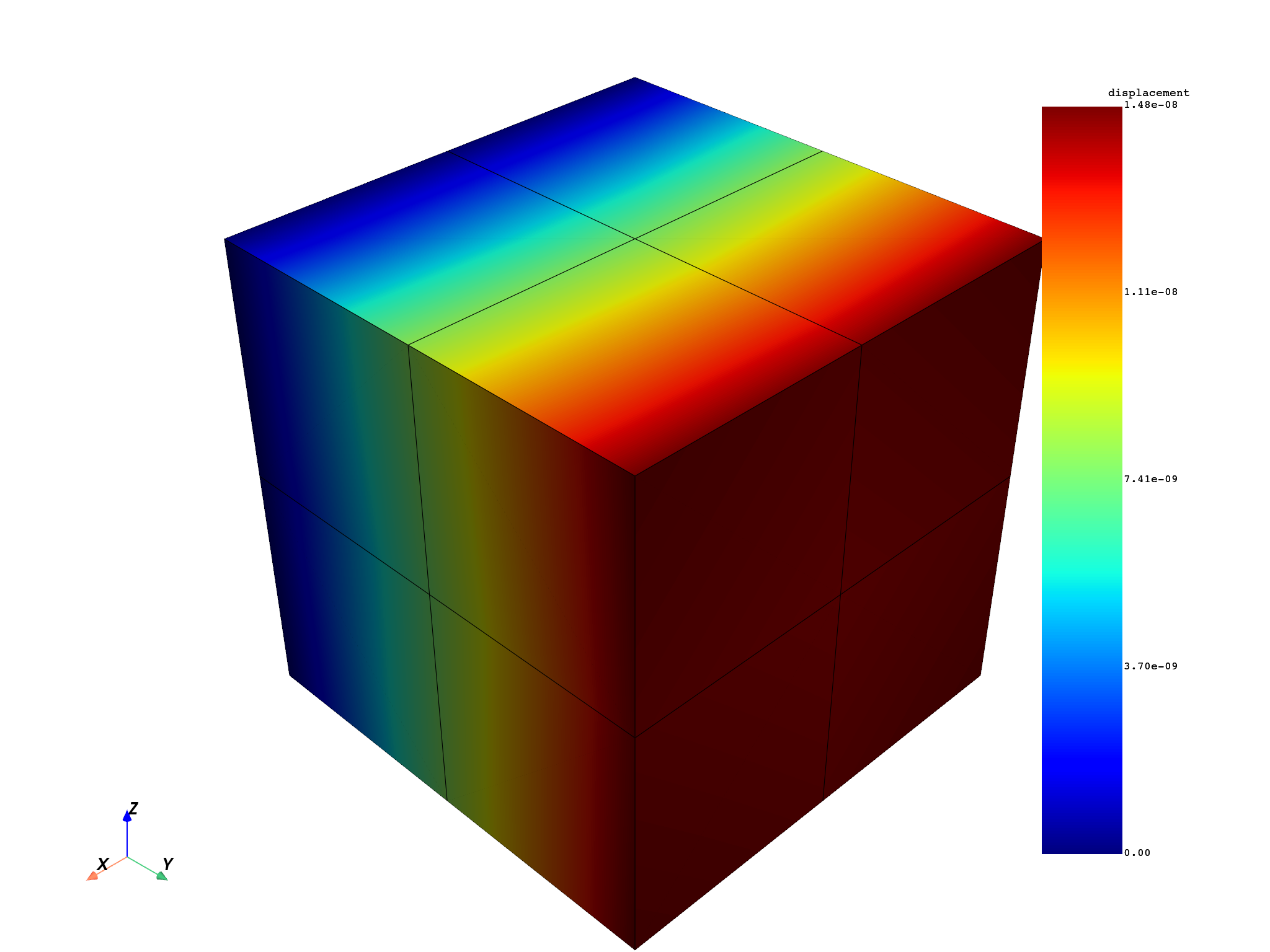## Get and plot stresses#

Request “XY” stress component averaged on nodes

```stress = simulation.stress_nodal(components="XY")
```

Print information

```print(stress._fc)
```
```DPF stress(s)Fields Container
with 1 field(s)
defined on labels: time

with:
- field 0 {time:  1} with Nodal location, 1 components and 81 entities.
```

Plot available stresses.

```stress._fc.plot()
```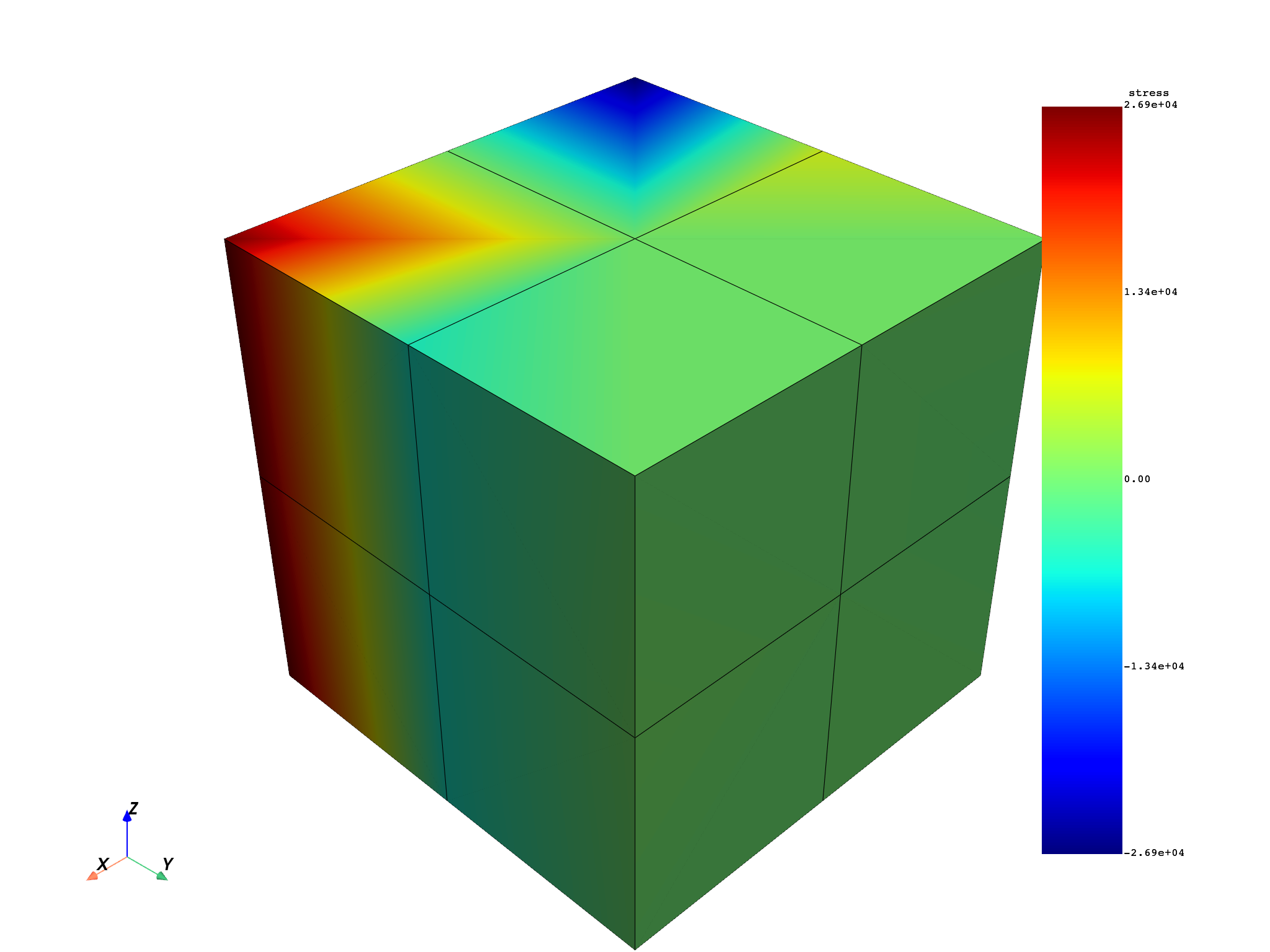## Get stresses at only 5 nodes#

Request stress only at the first 5 nodes using their IDs.

```stress_nodes = simulation.stress_nodal(node_ids=range(1, 6))
```

Print information

```print(stress_nodes._fc)
```
```DPF stress(s)Fields Container
with 1 field(s)
defined on labels: time

with:
- field 0 {time:  1} with Nodal location, 6 components and 5 entities.
```

Plot stresses

```stress_nodes._fc.plot()
```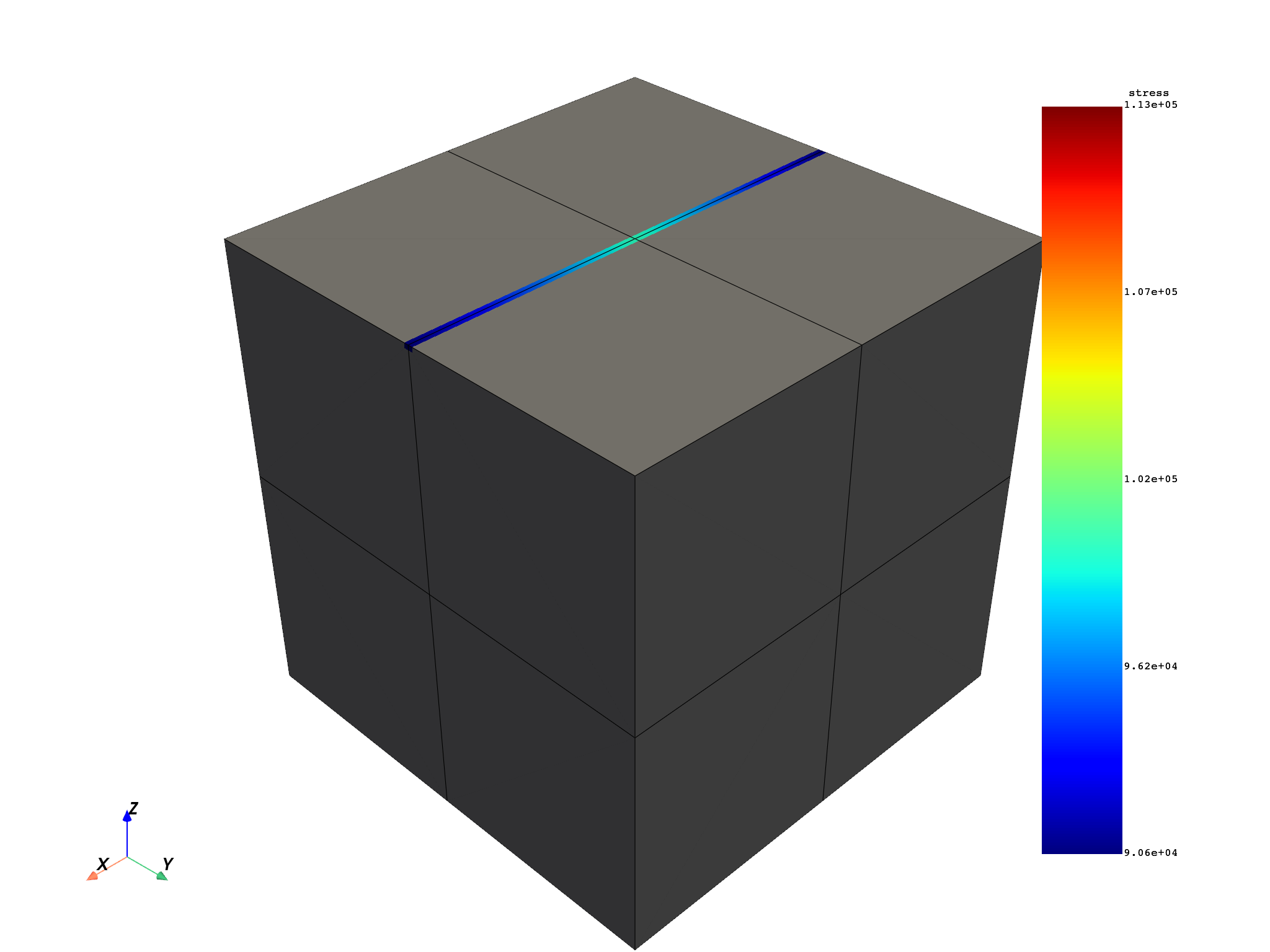## Get stresses in a named selection#

Get the name of the first named selection in the simulation

```ns = simulation.named_selections
# Request nodal stresses for this named selection
stress_named_sel = simulation.stress_nodal(named_selections=ns)
```

Print information

```print(stress_named_sel._fc)
```
```DPF stress(s)Fields Container
with 1 field(s)
defined on labels: time

with:
- field 0 {time:  1} with Nodal location, 6 components and 21 entities.
```

Plot stresses

```stress_named_sel._fc.plot()
```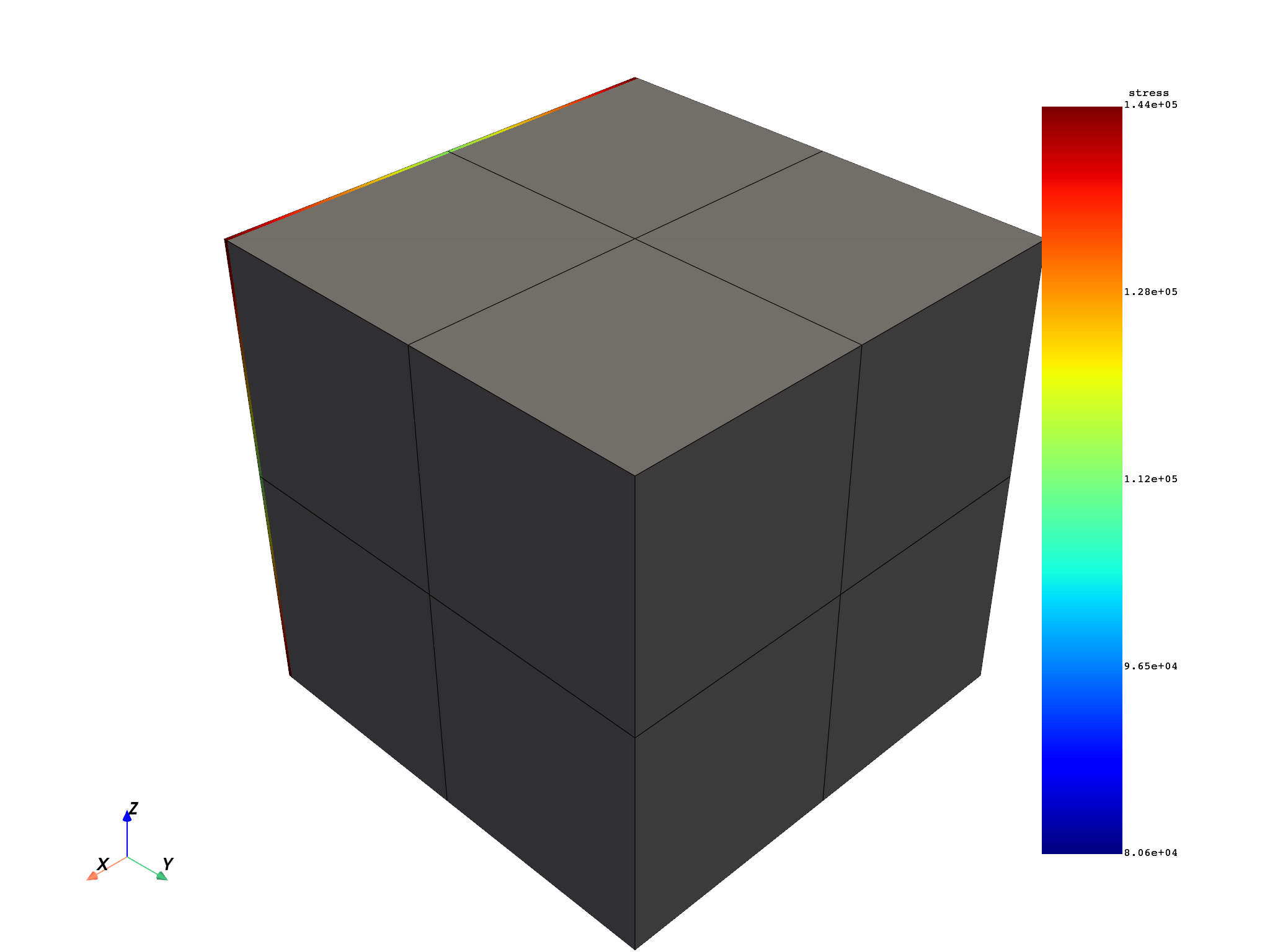## Get stresses in a few elements#

Request stress only for a few elements selected by their ID

```stress_elements = simulation.stress_nodal(element_ids=[1, 2, 3])
```

Print information

```print(stress_elements._fc)
```
```DPF stress(s)Fields Container
with 1 field(s)
defined on labels: time

with:
- field 0 {time:  1} with Nodal location, 6 components and 44 entities.
```

Plot stresses

```stress_elements._fc.plot()
```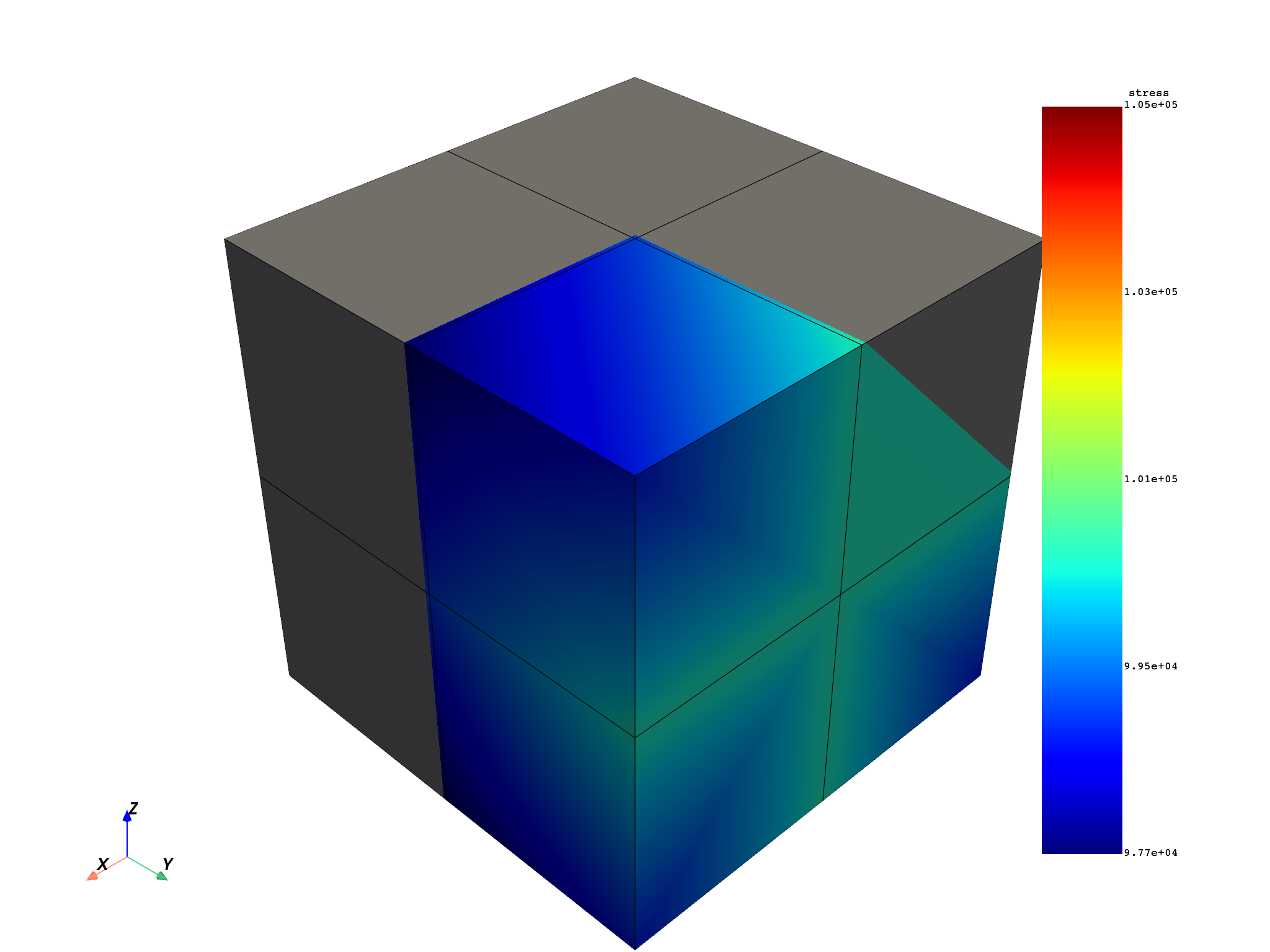## Get elemental stress and raw stresses#

Request elemental stresses and print information

```stress_elements = simulation.stress_elemental()
print(stress_elements._fc)
```
```DPF stress(s)Fields Container
with 1 field(s)
defined on labels: time

with:
- field 0 {time:  1} with Elemental location, 6 components and 8 entities.
```

Request raw stresses (“ElementalNodal”) and print information

```stress_raw = simulation.stress()
print(stress_raw._fc)
```
```DPF stress(s)Fields Container
with 1 field(s)
defined on labels: time

with:
- field 0 {time:  1} with ElementalNodal location, 6 components and 8 entities.
```

Total running time of the script: ( 0 minutes 2.939 seconds)

Gallery generated by Sphinx-Gallery